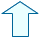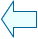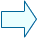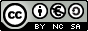Length Metres, litres, kilograms

3 . Length unit conversion.

We express the distance in kilometres when it is large, such as the distance between two towns.
We express the distance in millimetres when it is small, such as the thickness of a pencil.
The same measurement can be expressed in any unit but we have to use the most suitable unit. Besides, we sometimes have to add or subtract several measurements. In order to do this, they have to be expressed in the same unit, that is why it is fundamental to know how to convert units.

Esta unidad interactiva requiere la máquina virtual de Java J2RE.

The series of length units work in the same way as the units of our numeration system.
Each unit has a value ten times larger than the unit on the right and ten times smaller than the unit on the left.
You have to notice in which direction the unit jumps in order to move the decimal point in the same way.

 This activity shows a measurement expressed in a certain unit and on the right you will find the unit to do the conversion. Type the resulting number in the small window below. After typing the number hit Enter. If your answer is correct, this number will appear. If it isn't, try again.

When you click on Init, a different activity will appear. Do several conversions until you master unit conversion.Eduardo Barbero CorralSpanish Ministry of Education. Year 2007